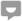# Basic Assumptions of Marginal Utility Analysis

Knoji reviews products and up-and-coming brands we think you'll love. In certain cases, we may receive a commission from brands mentioned in our guides. Learn more.
Basic Assumptions of Marginal Utility Analysis We shall first mention a few basic assumptions on which the marginal utility analysis is based. We shall see later how the marginal utility analysis has been criticized on the ground that the assumptions on

Basic Assumptions of Marginal Utility Analysis

We shall first mention a few basic assumptions on which the marginal utility analysis is based. We shall see later how the marginal utility analysis has been criticized on the ground that the assumptions on which it is based are unrealistic or invalid. The following are the main assumptions:—

(i) Cardinal Measurement of Utility. Marginal utility analysis assumes in the first place that utility can be measured and the exact measurement can be given by assigning definite numbers such as 1, 2, 3, etc. That is, it is assumed that utility is a quantifiable entity. This means that a person can express the satisfaction derived from the consumption of a commodity in quantitative terms. He can say, for instance, that for him the first unit of the commodity has utility equal to 10, the second unit 8, and so on. In this way, it is possible for a consumer to compare the utilities of different goods. If for example, fruit has for him utility 20 and sweets 10, then he can say, that for him the utility of fruits is double that of sweets. Utility is usually measured in imaginary units.

(ii) Utilities are Independent. Marginal utility analysis assumes that the utilities of different commodities are independent of one another. That is, the utility of one commodity does not in any way affect that of another. In other words, the satisfaction desired from the consumption of one good is the function of that good alone and is not affected by the consumption of another. It depends on the quantity consumed of one good and not of another. On this assumption, the total utility of all goods consumed by a consumer is simply the sum total of the separate utilities of all the goods consumed by a consumer. Thus, according to this assumption, the utilities of various goods are additive i.e. separate utilities of the various goods can be added to obtain the total sum of the utilities of all goods consumed.

(iii) Constant Marginal Utility of Money. Another important assumption of the marginal utility analysis is that the marginal utility of money remains constant even though the quantity of money with the consumer is diminished by the successive purchases made by him. It is assumed that while marginal utility of a commodity varies with the quantity of the commodity purchased, the marginal utility of money remains throughout the same as the quantity of the good purchased varies. This assumption becomes necessary because the marginal utility of a commodity is measured in terms of money. It is considered desirable that the measure itself should not keep changing. When a person purchases more of a good, the amount of money with him must diminish and the marginal utility of money must increase. But this variation in the marginal utility of money is ignored and it is assumed to remain constant throughout.

## 1 commentGuest
Posted on Jul 29, 2011